Geometry

# Volume: Level 3 Challenges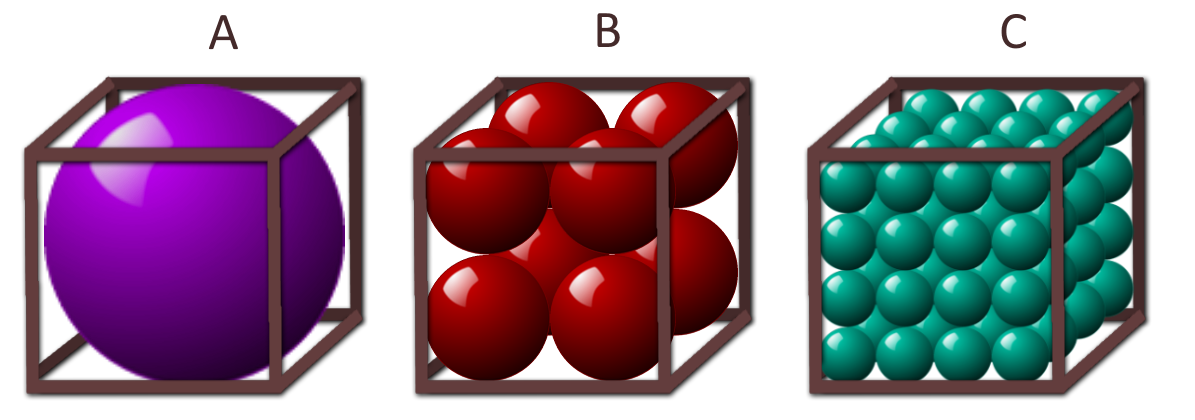Three identical tanks are shown above. The spheres in a given tank are the same size and packed wall-to-wall. If the tanks are filled to the top with water, then which tank would contain the most water?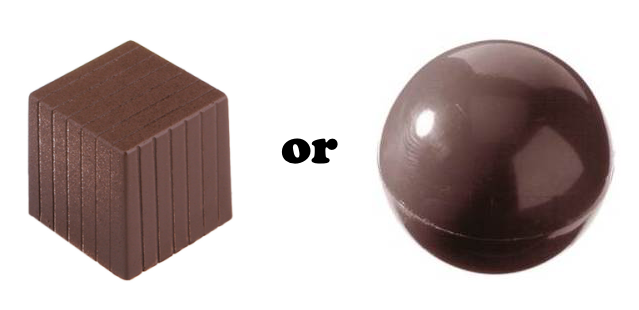A solid sphere of chocolate has a volume of $288\pi$. The chocolate is then melted down and reshaped into a solid cube. Assume no chocolate was wasted.

Did the sphere have a greater surface area or does the cube?

Useful equations:

• Volume of a sphere: $V=\frac{4}{3}\pi r^3$
• Volume of a cube: $V=a^3$
• Surface area of a sphere: $A=4\pi r^2$
• Surface area of a cube: $A=6a^2$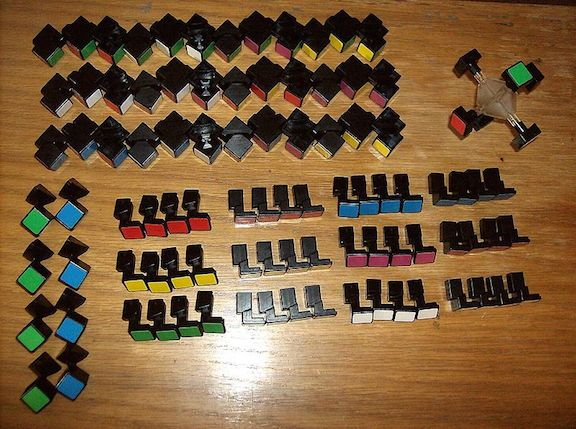How many cubes measuring 2 units on one side must be added to a cube measuring 8 units on one side to form a cube measuring 12 units on one side?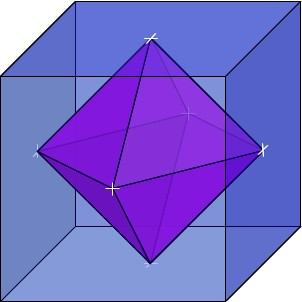What is the volume of the octahedron inside this $8 \text{ in}^3$ cube?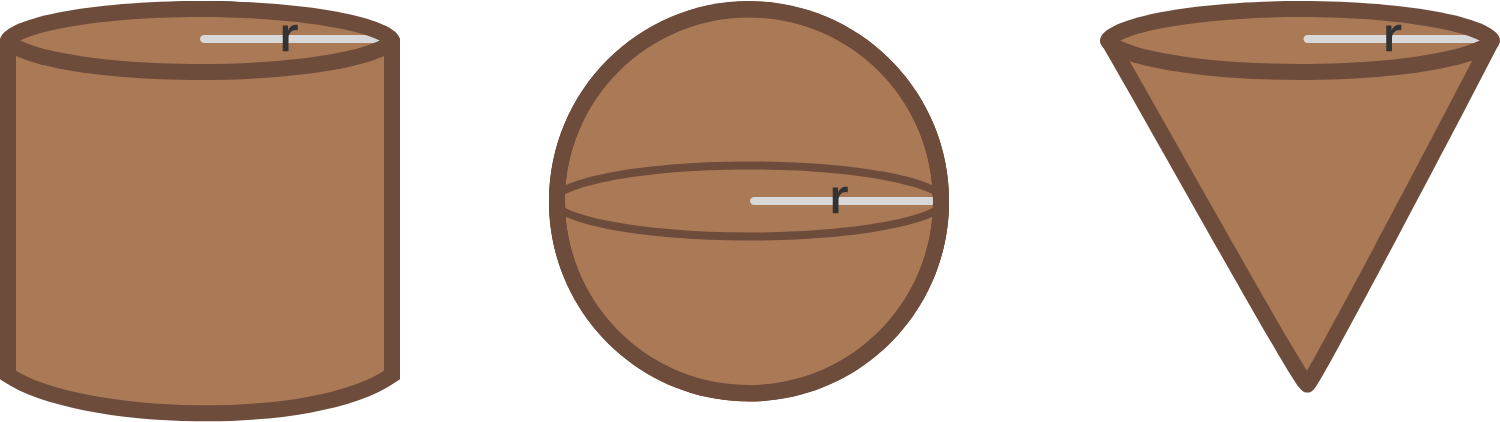A chocolate shop sells its products in 3 different shapes: a cylindrical bar, a spherical ball, and a cone. These 3 shapes are of the same height and radius, as shown in the picture. Which of these choices would give you the most chocolate?

$\text{ I. A full cylindrical bar } \hspace{.4cm} \text{ or } \hspace{.45cm} \text{ II. A ball plus a cone }$

×